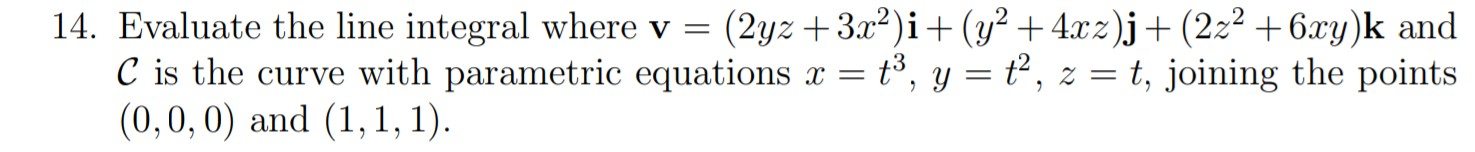# Question Solved1 Answer14. Evaluate the line integral where v = (2yz + 3x2)i + (y2 + 4xz)j + (222 + 6xy)k and C is the curve with parametric equations x = tº, y = 62, z = t, joining the points (0,0,0) and (1,1,1).Transcribed Image Text: 14. Evaluate the line integral where v = (2yz + 3x2)i + (y2 + 4xz)j + (222 + 6xy)k and C is the curve with parametric equations x = tº, y = 62, z = t, joining the points (0,0,0) and (1,1,1).
More
Transcribed Image Text: 14. Evaluate the line integral where v = (2yz + 3x2)i + (y2 + 4xz)j + (222 + 6xy)k and C is the curve with parametric equations x = tº, y = 62, z = t, joining the points (0,0,0) and (1,1,1).